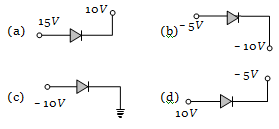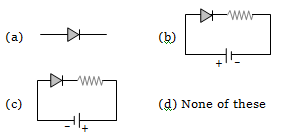Which one is reverse-biasedConcept Questions :-

Applications of PN junction
High Yielding Test Series + Question Bank - NEET 2020

Difficulty Level:

Which one is in forward biasConcept Questions :-

PN junction
High Yielding Test Series + Question Bank - NEET 2020

Difficulty Level:

The reason of current flow in P-N junction in forward bias is

(a) Drifting of charge carriers

(b) Minority charge carriers

(c) Diffusion of charge carriers

(d) All of these

Concept Questions :-

PN junction
High Yielding Test Series + Question Bank - NEET 2020

Difficulty Level:

The resistance of a reverse biased P-N junction diode is about
(a) 1 ohm                        (b) ${10}^{2}$ohm
(c) ${10}^{3}$ ohm                     (d) ${10}^{6}$ ohm

Concept Questions :-

PN junction

Difficulty Level:

Avalanche breakdown is due to

(a) Collision of minority charge carrier

(b) Increase in depletion layer thickness

(c) Decrease in depletion layer thickness

(d) None of these

Concept Questions :-

Energy band theory
High Yielding Test Series + Question Bank - NEET 2020

Difficulty Level:

Zener breakdown in a semi-conductor diode occurs when

(a) Forward current exceeds certain value

(b) Reverse bias exceeds certain value

(c) Forward bias exceeds certain value

(d) Potential barrier is reduced to zero

Concept Questions :-

Applications of PN junction
High Yielding Test Series + Question Bank - NEET 2020

Difficulty Level:

When forward bias is applied to a P-N junction, then what happens to the potential barrier ${V}_{\mathbit{B}}$, and the width of charge depleted region x

(a) ${V}_{\mathbit{B}}$ increases, x decreases

(b) ${V}_{\mathbit{B}}$ decreases, x increases

(c) ${V}_{\mathbit{B}}$ increases, x increases

(d) ${V}_{\mathbit{B}}$ decreases, x decreases

Concept Questions :-

PN junction
High Yielding Test Series + Question Bank - NEET 2020

Difficulty Level:

Function of rectifier is

(a) To convert ac into dc             (b) To convert dc into ac
(c) Both (a) and (b)                   (d) None of these

Concept Questions :-

Rectifier
High Yielding Test Series + Question Bank - NEET 2020

Difficulty Level:

When the P end of P-N junction is connected to the negative terminal of the battery and the N end to the positive terminal of the battery, then the P-N junction behaves like

(a) A conductor                       (b) An insulator
(c) A super-conductor              (d) A semi-conductor

Concept Questions :-

Applications of PN junction
High Yielding Test Series + Question Bank - NEET 2020

Difficulty Level:

A potential barrier of 0.50 V exists across a P-N junction. If the depletion region is $5.0×{10}^{-7}$m wide, the intensity of the electric field in this region is
(a)                       (b)
(c)                       (d)

Concept Questions :-

PN junction